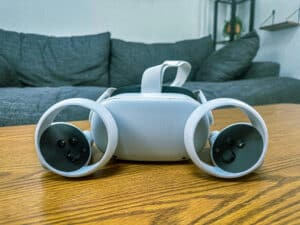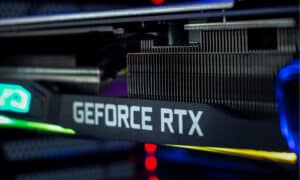Looking at a light bulb, a fridge, a television, or a phone charger, there’s one particular letter you’re likely to encounter again and again: the W. This stands for watts, which is a unit used to measure power. What, then, is the difference between a kilowatt hour vs a watt?

The two sound awfully similar, and it would make sense for them to be just as closely related in the grand scheme of things. However, the two serve two completely different purposes. What’s the real distinction between a kilowatt hour vs a watt, and what does each unit actually mean? Let’s elaborate.

## 5 Must-Know Facts About Power Consumption

1. Despite only accounting for less than 5% of the world’s population, the United States makes up for 26% of the world’s energy usage.
2. Of all the appliances and electronic devices in the home, more than 55% of an American home’s typical energy usage comes from the heating and cooling system. Another 10% comes from the home’s lighting.
3. Even in standby mode, our appliances continue to use about 85% of the energy they’d use when powered on. This is so that they can be ready and able to power on at a moment’s notice.
4. Though appliances and electronics grow more and more energy efficient each year, overall power consumption continues to grow at a rate of around 3% annually.
5. An energy-efficient washing machine tends to use around a third of the energy used by an older, less energy-efficient machine. However, washing clothes at the hottest temperature uses twice as much energy as a wash at a warm temperature.

## Kilowatt Hour vs Watt: What’s the Difference?

Now that we have a good understanding of the basic specs of the kilowatt hour vs watt, let’s spend some time elaborating on the differences between the two units. The best way to do this is to simply explain what each unit means, then compare the two based on these explanations. After that, we’ll review some real-world examples of each unit to further establish these key differences.

### Kilowatt Hour Explained

A kilowatt-hour is a composite unit of energy. Used to measure how many kilowatts (kW) are used over the course of one hour, a single kilowatt hour (or kWh) is equivalent to one kilowatt sustained over 60 minutes.

When expressed a different way, using the joule (J) — the most commonly used unit of energy within the International System of Units (SI) — a single kilowatt hour is equivalent to 3,600,000 joules, 3,600 kilojoules (kJ), or 3.6 megajoules (MJ). In your daily life, you’re most likely to encounter this particular unit of energy on a power bill.

A kilowatt hour depends on a couple of factors: how many watts the appliances in your home use, for one, combined with how often you use the appliances when powered on. If your appliance used one kilowatt and it was powered on for one hour, that measurement would be 1 kWh.

With that being said, the abbreviation “kWh” is not to be confused with “kW/h,” which refers to kilowatts per hour. This latter abbreviation suggests a measurement of “kilowatts per hour,” which is not an actual measurement at all. It’s a misnomer.

In the world of utilities, customers are typically sold electrical energy in terms of kilowatt hours. In this usage, the device’s power consumption (measured in kilowatts) is multiplied by the number of hours the device was in operation. That total is called kilowatt hours.

The power company then multiplies the kilowatt hours by a certain price per kilowatt hour. This calculation results in the total cost it took to run the electrical device. Repeat for every appliance consuming power in the home over the course of the billing period and, generally speaking, you’ll have that month’s power bill.

### Watt Explained

Here’s the most important thing to understand about the difference between kilowatt hour vs watt: a kilowatt hour is a unit of energy, whereas a watt is a unit of power. When measured by the International System of Units (SI), a watt is equivalent to one joule a second (or j/s).

(Two watts would be two joules per second, 10 watts would equal 10 joules, 1,000 watts would be 1 kW which would be 1,000 joules per second, and so on.) While the kWh is used to measure how many kilowatts are sustained in an hour, a watt quantifies the rate that energy is transferred.

The math is actually pretty simple. When the velocity of an object is maintained at a constant of one meter a second working against a consistent opposite force maintained at one newton, you’re looking at a rate of work of one watt.

In physics, it gets even simpler: the watt represents the rate electrical work is done. When an electrical current of one ampere, or amp (A), flows across an electrical potential difference (or electric pressure) of one volt (V), you’ve got a watt. In other words, a watt equals voltage times amperage. In abbreviated terms, W = V x A.

The watt derives its name from James Watt, a Scottish inventor, chemist, and electrical engineer. Watts had the honor of becoming the unit’s namesake after a long line of units being named after other leading physicists in the industry.

It was proposed by another electrical engineer, one C. William Siemens, in 1882. However, it wasn’t standardized until 1908 — another 20 years after the initial proposal from Siemens. In the years that followed, various units for multiple watts were adopted. These included the kilowatt, the megawatt, the gigawatt, and so on.

## Kilowatt Hour vs Watt: Real-World Examples

After explaining the differences in a kilowatt hour vs watt, it helps to lock in these dissimilarities through the use of some real-world examples. Where might you see a kilowatt hour in your daily life? And what about a watt by comparison? Let’s elaborate on the two below.

### Kilowatt Hour Examples

1. A bright 100-watt light bulb left on for ten hours is equivalent to one kilowatt-hour.
2. A 150-watt television left on for about six and a half hours is equivalent to one kilowatt-hour.
3. The average American household uses around 900 kWh per month.
4. Typical prices per kWh can vary tremendously from state to state. Sometimes, it’s a difference of as much as three times. For instance, in the Midwest, prices might be closer to a dime per kWh. But, on the East Coast, the price might be closer to 30 cents per kWh.
5. Baking in an oven uses around three kWh. Frying, by comparison, uses closer to two kWh.

### Watt Examples

1. A 100 W lightbulb powered on for one hour uses the same amount of watts as a 40 W lightbulb would in two and a half hours, or a 50 W bulb in two hours.
2. Ten 100-watt bulbs left on for one hour would use 1,000 watts. This amount of power is equivalent to one kWh of energy.
3. Watts are the electrical equivalent of horsepower. With this in mind, a single unit of horsepower is equivalent to 745 watts of power.
4. A washing machine tends to use between 350 and 500 watts. A dryer, by comparison, uses as much as 1,800 to 5,000 watts.
5. While resting, a person exerts 100 watts of power. While sprinting, a person exerts as much as 2,000 watts or more.

## Kilowatt Hour vs Watt: Which One Is Best For You?

The debate between kilowatt hour vs watt really is as simple as the difference between energy and power. In basic terms, energy is a person or an object’s capacity to do some sort of physical activity or work. Power, by comparison, is the rate an activity or work is done.

In other words, energy is an ability, while power is a measurement of the ability. The same is true of the kilowatt hour vs watt. A 100 W light bulb needs 100 watts of power to turn on, which means its energy would be the length of time the bulb is using that power.

So, that said, the kilowatt-hour measurement is best for those trying to gauge the total usage of energy. The watt, on the other hand, is best for those trying to gauge the total usage of power. An interesting way to think of it is that you can measure watts without ever considering kilowatt hours, but you can’t measure kilowatt hours without first measuring watts.

No one unit is better than the other in this respect. They need each other in order to make the equations make sense. (For the record, computers use around 50 W, which means a single Google search uses about 0.0003 kWh of energy.)

## Kilowatt Hour vs Watt: What’s the Difference? FAQs (Frequently Asked Questions)

What is a watt?

A watt (W) is a unit of power used to measure the rate energy is transferred. It’s a staple of the International System of Units (SI) and is equivalent to one joule per second (or j/s).

What is a kilowatt hour?

A kilowatt hour (kWh) is a unit of energy used to measure the number of kilowatts used over the course of one hour. While used outside of the International System of Units, it does have an equivalent in SI units: 3.6 megajoules (MJ). This unit of energy is commonly used on billing statements from electric companies.

How many watts are in a kilowatt hour?

In simple terms, a single kilowatt hour is equivalent to a thousand watts used continuously for one hour.

How many kilowatt hours are in one watt?

While watts can be converted to kilowatt hours, the inverse cannot be true. A unit of power needs a unit of energy in order to describe the amount of energy used. However, a unit of energy is not measured in power, so the two are not exactly comparable. It would be like asking “How many miles an hour are in a meter?” It just doesn’t compute.

What appliance uses the most power?

The heating and cooling system is the appliance that uses the most power in the home. On average, it accounts for more than 50% of the home’s electricity usage.

## More from History-Computer##### Meta Quest 2 vs Oculus Quest 2: Is There a Difference?##### Nvidia RTX 3080 vs 3080-Ti: Full Comparison With Specs, Price, and More##### The 10 Largest Chip Manufacturers in the World and What They Do##### The 10 Largest and Most Important Battery Companies in the World
• Energy Lens Available here: https://www.energylens.com/articles/kw-and-kwh
• EVESCO Available here: https://www.power-sonic.com/blog/kw-vs-kwh-explained/
• Engineering Mindset Available here: https://theengineeringmindset.com/kilowatt-hours-kwh-explained/
• TechTarget Available here: https://www.techtarget.com/whatis/definition/watt
• The Spruce Available here: https://www.thespruce.com/the-difference-between-watts-vs-volts-4767057